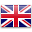English

# The underreported model

The phenomenon of underreporting in the context of biomedical research has been widely studied, at least since the works of Bernard et. al. in 2011 . A slightly more recent reference is Fernández-Fontelo et. al. in 2016 , where a very simple mechanism is proposed to model the phenomenon. Let us consider a hidden process (the real daily number of positive cases, which is not observable since the entire population of interest is not tested daily)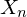with an autoregressive structure of order 1, for integer data and with Poisson innovations, Po- INAR (1):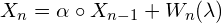where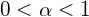is a fixed parameter,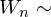Poisson(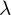), i.i.d., independent of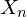and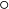is the operator of thinning binomial: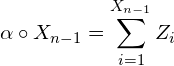con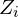variables aleatorias i.i.d. con distribución de Bernoulli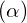.
El proceso INAR(1) es una cadena de Markov homogénea con probabilidades de transición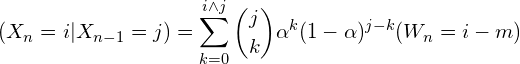La esperanza y varianza del operador de thinning binomial son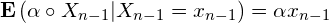y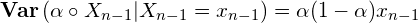#### Un esquema simple de infra-reporte

The underreporting phenomenon is modeled assuming that the observed counts have the following form:

(1)where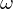yrepresent the frequency and intensity of the underreported process. That is, for each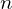, a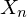can be observed with a probability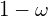, and a-thinning ofwith probability, regardless of previous. Therefore, what is observed (the reported counts) is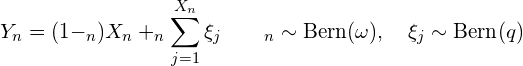This model will surely have to be modified to make bothand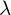are dependent on time.

#### Model properties

The expectation and variance of the INAR (1)process with Poisson innovations () is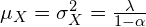. The autocovariances and autocorrelations arerespectively. On the other hand,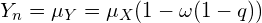.  The autocovariance from the observed process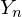is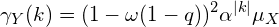Thus, the autocorrelation functionis a multiple of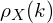The shape of the expected valuealso allows to obtain predictions forhaving seen until.

#### Parameter estimation

The marginal distribution ofis a mixture of two Poission distributions.

(2)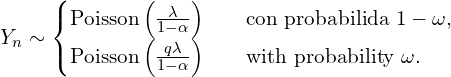Whenthe distribution of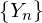Poisson zero-inflated. From the mixture we can have preliminary estimates for the parameters that will later be used in a calculation of the maximum likelihood estimators. The plausibity ofis very complicated,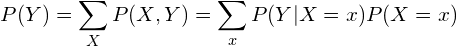and to calculated the forward algorithm ~\cite{lystig02} is widely used in the context of hidden Markov models. The probabilities of forward are

(3)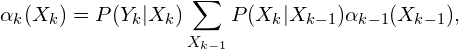con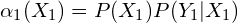. Therefore the plausibity is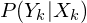ythese are the so-called emission and transition probabilities. Transition probabilities are calculated as follows:

(4)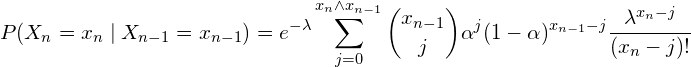and the emision ones

(5)#### Rebuilding the hidden chainTo reconstruct the hidden series, the \textbf{Viterbi}  algorithm is used as described previously.

The main idea to reconstruct the latent chain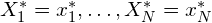which maximizes the probability of the latent process given the observations, assuming that the parameters are known.

#### Bibliografía

 Bernard H,Werber D, Hoehle M. Estimating the under-reporting of norovirus illness in Germany utilizing enhanced awareness of diarrhoea during a large outbreak of Shiga toxin-producing E. coli O104: H4 in 2011-a time series analysis. BMC
Infectious Diseases 2014; 14:116. DOI:10.1186/1471-2334-14-116.

 Fan, C. et al. Prediction of Epidemic Spread of the 2019 Novel Coronavirus Driven by Spring Festival Transportation in China: A Population-Based Study. Int. J. Environ. Res. Public Health 17, (2020).

 Fernández-Fontelo A., Cabaña A., Puig P., Moriña D. Under-reported data analysis with INAR-hidden Markov chains
Statistics in Medicine (2016), vol. 35, Issue 26, 4875-4890.

 Fernández‐Fontelo, A., Cabaña, A., Joe, H., Puig, P. & Moriña, D. Untangling serially dependent underreported count data for gender‐based violence. Stat. Med. 38, 4404–4422 (2019).

 Moriña, D., Fernández-Fontelo, A., Cabaña, A.  & Puig, P. New statistical model for misreported data with application to current public health challenges. Submitted to Statistical Methods in Medical Research, (2020).

 Moriña, D., Fernández-Fontelo, A., Cabaña, A., Puig, P., Monfil, L., Brotons, M. & Diaz, M. Quantifying the underreporting of genital warts cases. Submitted to the European Journal of Epidemiology (2020).

 Kermack W. O., McKendrick A. G. (1927). A Contribution to the Mathematical Theory of Epidemics. Proceedings of the Royal Society A. 115 (772): 700–721.

 T.C. Lystig, J.P. Hughes (2002),  Exact computation of the observed information matrix for hidden Markov models, J. of Comp.and Graph. Stat.

 Viterbi, A.J. (1967), Error bounds for convolutional codes and an asymptotically optimum decoding algorithm. IEEE Transactions on Information Theory,  13, 260D269.